Select Page

# Maths Probability CBSE 12 Science MCQ in English Answers

Maths Probability CBSE 12 Science MCQ in English Answers to enable students to get Answers in a narrative video format for the specific question.

Expert Teacher provides Maths Probability CBSE 12 Science MCQ English Answers. This video solution will be useful for students to understand how to write an answer in exam in order to score more marks. This teacher uses a narrative style for a question from Probability not only to explain the proper method of answering question, but deriving right answer too.

Please find the question below and view the Answer in a narrative video format.

Question:

## Similar Questions from CBSE, 12th Science, Maths, Probability

Question 1 : A and B throw a pair of dice alternately. A wins the game if he gets a total of 7 and B wins the game if he gets a total of 10. If A starts the game, the find the probability that B wins.   (View Answer Video)

Question 2 : If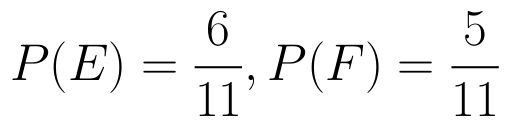and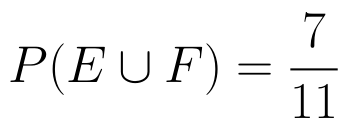then find P(E / F). (View Answer Video)

Question 3 : Three cards are drawn without replacement from a pack of 52 cards. Find the probability that the cards drawn are king, queen and jack respectively.

Question 4 : A die is thrown 6 times. If 'getting an odd number' is a 'success', what is the probability of obtaining:
atleast 5 successes?

Question 5 : If P(A)=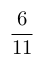, P(B)=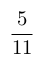and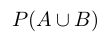=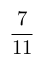, find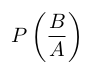. (View Answer Video)

### Continuity and Differentiability

Question 1 :  Find the second order derivative of the function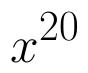. (View Answer Video)

Question 2 : Differentiate w.r.t.x the function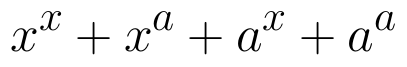, for some fixed a > 0 and x > 0. (View Answer Video)

Question 3 : Differentiate the function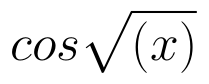with respect to x. (View Answer Video)

Question 4 : Findfor the function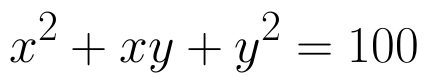. (View Answer Video)

Question 5 : Differentiate the function w.r.t.x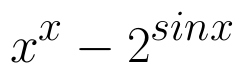. (View Answer Video)

### Differential Equations

Question 1 : Write the differential equation representing the curve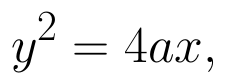where a is an arbitrary constant. (View Answer Video)

Question 2 : Obtain the differential equation of the family of circles passing through the points (a, 0) and (-a, 0). (View Answer Video)

Question 3 : Solve the differential equation: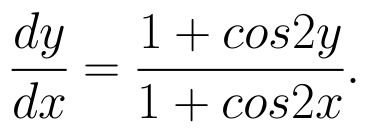(View Answer Video)

Question 4 : If y(x) is a solution of the differential equation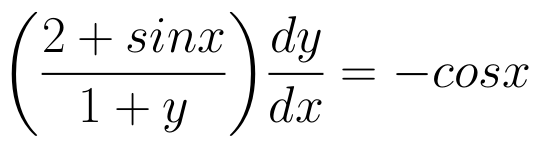and y(0) = 1, then find the value of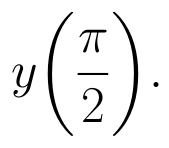(View Answer Video)

Question 5 : Write the differential equation obtained by eliminating the arbitrary constant C in the equation representing the family of curves xy = C cos x. (View Answer Video)

### Vector Algebra

Question 1 : Find the vector quantities from the following:
(i) Time period          (ii) Distance          (iii) Force
(iv) Velocity               (v) Work done
Question 2 : Find the magnitude of two vectors a and b having the same magnitude and such that the angle between them is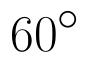and their scalar product is 1/2. (View Answer Video)
(i) 10 kg          (ii) 2 m north-west          (iii)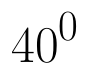(iv) 40 W         (v)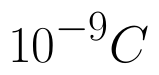(vi)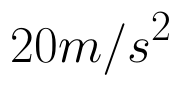(View Answer Video)
Question 4 : Find a unit vector parallel to the sum of vectors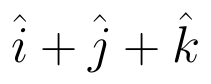and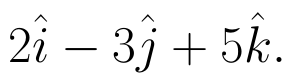(View Answer Video)
Question 5 :  Write a unit vector in the direction of the sum of vectors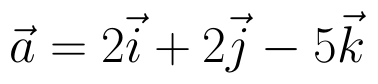and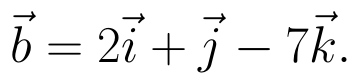(View Answer Video)